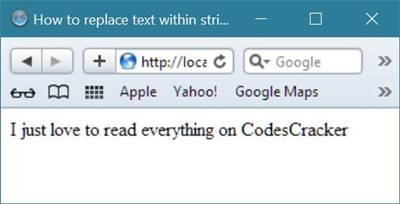# PHP Strings

Strings in PHP, are basically a sequence of characters such as "i am string", "hello world", "hello php strings", "strings", etc.

## PHP Strings Example

Here is an example on string in PHP.

```<html>
<title>PHP String Example</title>
<body>
<?php
\$string_var1 = "Codes";
\$string_var2 = "Cracker";
echo \$string_var1;
echo \$string_var2;
?>
</body>
</html>```

Below is the sample output of the above string example code in PHP.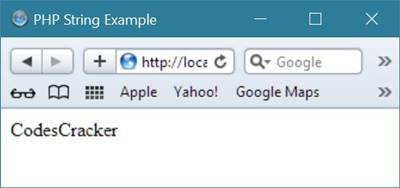Below are the list of some more examples based on strings in PHP. Let's first start with, how to find the length of the string in PHP.

## Get Length of String in PHP

To get the length of a string in PHP, use a function named strlen(). This function returns the length (total number of character) of the string.

Here is an example, uses strlen() function to fing the length of the string in PHP:

```<!DOCTYPE html>
<html>
<title>How to find length of any string in PHP ?</title>
<body>
<?php
\$string_var = "I am string in PHP";
\$string_length = strlen(\$string_var);
// spaces also count in string length
echo "Length of String = ".\$string_length;
?>
</body>
</html>```

As you can see from the above PHP code that shows how to find length of any string, first we have initialized a string "I am string in PHP" to the variable \$string_var. Now we have initialized total length of the string contained in the variable, \$string_var to the variable \$string_length using the function strlen(). And at last we have printed the length of the string as output as shown in the following sample output.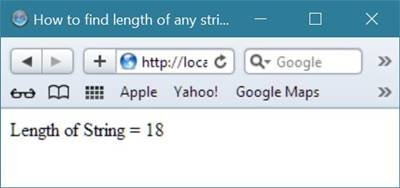## Count Number of Words in String in PHP

To count total number of words present in the string in PHP, use a function called str_word_count(). This function counts total number of words present in the string in PHP. Here is an example, uses str_word_count() function to count words in string in PHP:

```<!DOCTYPE html>
<html>
<title>How to count total number of words present in String in PHP ?</title>
<body>
<?php
\$string_var = "Hello sir, you are learning string in PHP on CodesCracker";
\$total_word_in_string = str_word_count(\$string_var);
echo "Total word present in string = ".\$total_word_in_string;
?>
</body>
</html>```

Since there are 10 words in the string, therefore the output will display 10 string as shown in the given figure.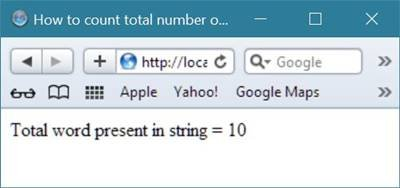## Reverse String in PHP

To reverse a string in PHP, use a function named strrev(). This function reverses a string in PHP. Here is an example:

```<!DOCTYPE html>
<html>
<title>How to reverse any string in PHP ?</title>
<body>
<?php
\$string_var = "Hello sir, you are learning string in PHP on CodesCracker";
\$string_reverse = strrev(\$string_var);
echo "Reverse of string: <b>".\$string_var."</b> will be<hr/>";
echo \$string_reverse;
?>
</body>
</html>```

It will display the following result: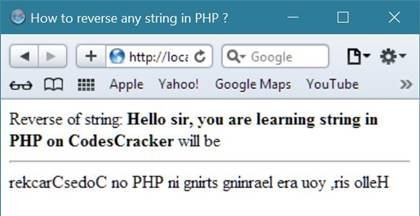## Search for Specific Text within String in PHP

To search for the specific text present in the string in PHP, then use a function named strpos(). This function searches for a specific text within the string.

In case, if a match is found, then the function returns the character's position of the first match. And if no match is found, then it will return FALSE. Here is an example searches for the text "world" in the string "Hello World!, This is PHP":

```<!DOCTYPE html>
<html>
<title>How to search for specific text within string in PHP ?</title>
<body>
<?php
\$string_var = "Hello World!, This is PHP";
\$find_world = strpos(\$string_var, "World");
\$find_codes = strpos(\$string_var, "Codes");
\$find_is = strpos(\$string_var, "is");
if(\$find_world)
{
echo "World is at position: ".\$find_world;
echo "<br/>";
}
if(\$find_codes)
{
echo "Codes is at position: ".\$find_codes;
echo "<br/>";
}
if(\$find_is)
{
echo "is is at position: ".\$find_is;
echo "<br/>";
}
?>
</body>
</html>```

It will produce the following result: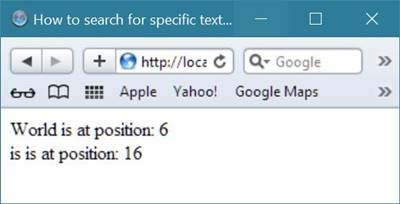## Replace Text within String in PHP

To replace text within string in PHP, then use a function named str_replace(). This function replaces some characters with some other characters in the string in PHP. Here is an example, replaces the text "world" with "PHP"

```<!DOCTYPE html>
<html>
<title>How to replace text within string in PHP ?</title>